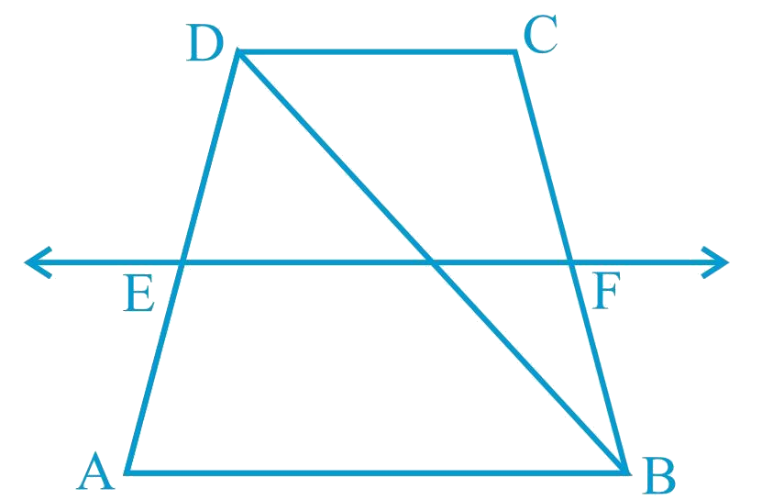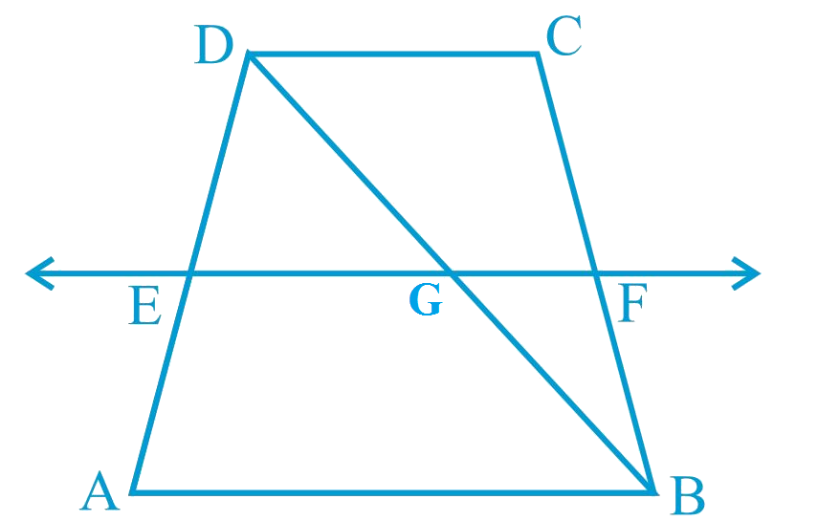In the verge of coronavirus pandemic, we are providing FREE access to our entire Online Curriculum to ensure Learning Doesn't STOP!

# Ex.8.2 Q4 Quadrilaterals Solution - NCERT Maths Class 9

Go back to  'Ex.8.2'

## Question

$$ABCD$$ is a trapezium in which $$AB$$ $$\parallel$$ $$DC$$, $$BD$$ is a diagonal and $$E$$ is the mid - point of $$AD$$. $$A$$ line is drawn through $$E$$ parallel to $$AB$$ intersecting $$BC$$ at $$F$$ (see the given figure). Show that $$F$$ is the mid-point of $$BC$$.Video Solution
Ex 8.2 | Question 4

## Text Solution

What is known?

ABCD is a trapezium in which $$AB DC, BD$$ is a diagonal and $$E$$ is the mid - point of $$AD.$$ A line is drawn through $$E$$ parallel to $$AB$$ intersecting $$BC$$ at $$F.$$

What is unknown?

How we can show that F is the mid-point of $$BC.$$

Reasoning:

By converse of mid-point theorem, we know that a line drawn through the mid-point of any side of a triangle and parallel to another side, bisects the third side.

Steps:

Let $$EF$$ intersect $$DB$$ at $$G$$.By converse of mid-point theorem, we know that a line drawn through the mid-point of any side of a triangle and parallel to another side, bisects the third side.

In $$\Delta {ABD,}$$

$$EF$$ $$\parallel$$ $$AB$$ and $$E$$ is the mid-point of $$AD$$.

Therefore, $$G$$ will be the mid-point of $$DB$$.

As  $$EF$$ $$\parallel$$ $$AB$$ and $$AB$$ $$\parallel$$ $$CD$$,

$$\therefore$$  $$EF$$ $$\parallel$$ $$CD$$ (Two lines parallel to the same line are parallel to each other)

In $$\Delta {BCD,}$$ $$GF$$ $$\parallel$$ $$CD$$ and $$G$$ is the mid-point of line $$BD$$. Therefore, by using converse of mid-point theorem, $$F$$ is the mid-point of $$BC$$.

Video Solution